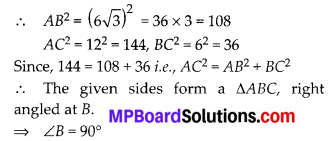# MP Board Class 10th Maths Solutions Chapter 6 Triangles Ex 6.5

In this article, we will share MP Board Class 10th Maths Book Solutions Chapter 6 Triangles Ex 6.5 Pdf, These solutions are solved subject experts from the latest edition books.

## MP Board Class 10th Maths Solutions Chapter 6 Triangles Ex 6.5

Question 1.
Sides of triangles are given below. Determine which of them are right triangles. In case of a right triangle, write the length of its hypotenuse.
(i) 7 cm, 24 cm, 25 cm
(ii) 3 cm, 8 cm, 6 cm
(iii) 50 cm, 80 cm, 100 cm
(iv) 13 cm, 12 cm, 5 cm
Solution:
(i) The sides are : 7 an, 24 cm, 25 cm
Here, (7 cm)2 = 49 cm2
(24 cm)2 = 576 cm2
(25 cm)2 = 625 cm2
∵ (49 + 576)cm2 = 625 cm2
∴ It is a right triangle.
Hypotenuse = 25 cm.

(ii) The sides are: 3 cm, 8 cm, 6 cm
Here, (3 cm)2 = 9 cm2
(8 cm)2 = 64 cm2
(6 cm)2 = 36 cm2
∵ (9 + 36) ≠ 64 cm2
∴ It is not a right triangle.

(iii) The sides are : 50 cm, 80 cm, 100 cm
Here, (50 cm)2 = 2500 cm2
(80 cm)2 = 6400 cm2
(100 cm)2 = 10000 cm2
∵ (2500 + 6400) cm2 ≠ 10000 cm2
∴ It is not a right triangle.

(iv) The sides are : 13 cm, 12 cm, 5 cm
Here, (13 cm)2 = 169 cm2
(12 cm)2 = 144 cm2
(5 cm)2 = 25 cm2
∵ (144 + 25)cm2 = 169 cm2
∴ It is a right triangle.
Hypotenuse = 13 cm.Question 2.
PQR is a triangle right angled at P and M is a point on QR such that PM ⊥ QR. Show that PM2 = QM.MR.
Solution:
In ∆QMP and ∆QPR,
∠QMP = ∠QPR [Each 90°]
∠Q = ∠Q [Common]
⇒ ∆QMP ~ ∆QPR …. (1) [AA similarity]
Again, in ∆PMR and ∆QPR,
∠PMR = ∠QPR [Each = 90°]
∠R = ∠R [Common]
⇒ ∆PMR ~ ∆QPR …… (2) [AA similarity]
From (1) and (2), we have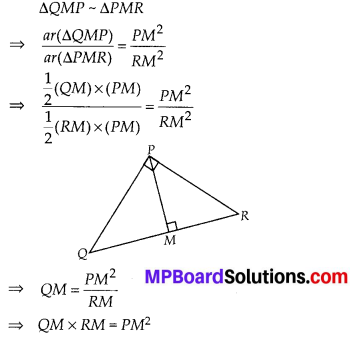Question 3.
In the figure, ABD is a triangle, right angled at A and AC ⊥ BD.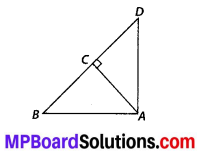Show that
(i) AB2 = BC.BD
(ii) AC2 = BC.DC
Solution: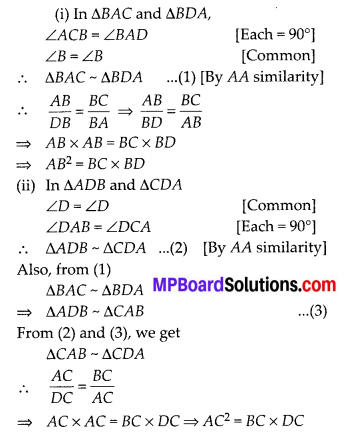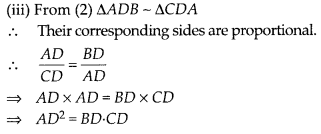Question 4.
ABC is an isosceles triangle right angled at C. Prove that AB2 = 2AC2.
Solution:
We have, right ∆ABC such that ∠C = 90° and AC = BC.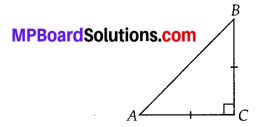∴ By Pythagoras theorem, we have AB2 = AC2 + BC2 = AC2 + AC2 = 2AC2
[∵ BC = AC (given)]
Thus, AB2 = 2AC2

Question 5.
ABC is an isosceles triangle with AC = BC. If AB2 = 2AC2, prove that ABC is a right triangle.
Solution:
We have, an isosceles AABC such that BC = AC.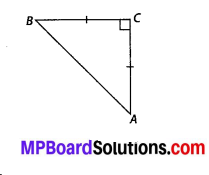Also, AB2 = 2AC2
∴ AB2 = AC2 + AC2
But AC =BC
∴ AB2 = AC2 + BC2
∴ Using the converse of Pythagoras theorem, ∠ACB = 90°
f.e., ∆ABC is a right angled triangle.Question 6.
ABC is an equilateral triangle of side 2a. Find each of its altitudes.
Solution:
In equilateral triangle, altitude bisects the base.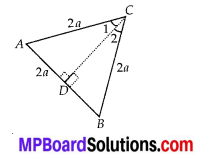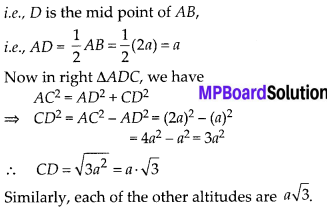Question 7.
Prove that the sum of the squares of the sides of a rhombus is equal to the sum of the squares of its diagonals.
Solution:
Let us have a rhombus ABCD.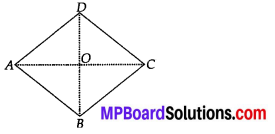∵ Diagonal of a rhombus bisect each other at right angles.
∴ OA = OC and OB = OD
Also, ∠AOB = ∠BOC [Each = 90°]
And ∠COD = ∠DOA [Each = 90°]
In right ∆AOB, we have,
AB2 = OA2 + OB2 …… (1)
[Using Pythagoras theorem]
Similarly, in right ∆BOC,
BC2 = OB2 + OC2 …… (2)
In right ∆COD,
CD2 = OC2 + OD2 …… (3)
In right ∆AOD,
DA2 = OD2 + OA2 ……. (4)
Adding (1), (2), (3) and (4)
AB2 + BC2 + CD2 + DA2
= [OA2 + OB2] + [OB2 + OC2] + [OC2 + OD2] + [OD2 + OA2]
= 2OA2 + 2OB2 + 2 OC2 + 2OD2 = 2[OA2 + OB2 + OC2 + OD2]
= 2[OA2 + OB2 + OA2 + OB2]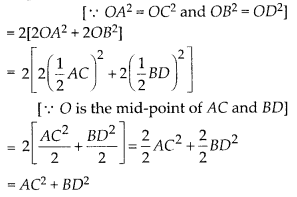Thus, sum of the squares of the sides of a rhombus is equal to the sum of the squares of its diagonals.

Question 8.
In the figure, O is a point in the interior of a triangle ABC, OD ⊥ BC, OE ⊥ AC and OF ⊥ AB. Show that
(i) OA2 + OB2 + OC2 – OD2 – OE2 – OF2 = AF2 + BD2 + CE2
(ii) AF2 + BD2 + CE2 = AE2 + CD2 + BF2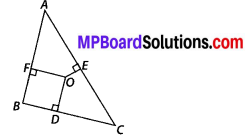Solution:
We have a point in the interior of a ∆ABC such that
OD ⊥ BC, OE ⊥ AC and OF ⊥ AB.
(i) Let us join OA, OB and OC.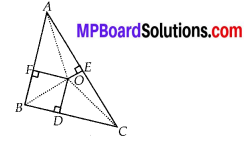In right ∆OAF, by Pythagoras theorem
OA2 = OF2 + AF2 …(1)
Similarly, from right triangle ODB and OEC, we have
OB2 = BD2 + OD2, …(2)
and OC2 = CE2 + OE2 …(3)
Adding (1), (2) and (3), we get OA2 + OB2 + OC2
= (AF2 + OF2) + (BD2 + OD2) + (CE2 + OE2)
⇒ OA2 + OB2 + OC2
= AF2 + BD2 + CE2 + (OF2 + OD2 + OE2)
⇒ OA2 + OB2 + OC2 – (OD2 + OE2 + OF2)
= AF2 + BD2 +CE2
⇒ OA2 + OB2 + OC2 – OD2 – OE2 – OF2
= AF2 + BD2 + CE2

(ii) In right triangle OBD and triangle OCD, by Pythagoras theorem:
OB2 = OD2 + BD2 and OC2 = OD2 + CD2
⇒ OB2 – OC2 = OD2 + BD2 – OD2 – CD2
⇒ OB2 – OC2 = BD2 – CD2 ….. (1)
Similarly, we have
OC2 – OA2 = CE2 – AE2 …… (2)
and OA2 – OB2 = AF2 – BF2 ….. (3)
Adding (1), (2) and (3), we get (OB2 – OC2) + (OC2 – OA2) + (OA2 – OB2) = (BD2 – CD2) + (CE2 – AE2) + (AF2 – BF2)
⇒ 0 = BD2 + CE2 + AF2 – (CD2 + AE2 + BF2)
⇒ BD2 + CE2 + AF2 = CD2 + AE2 + BF2
or AF2 + BD2 + CE2 = AE2 + BF2 + CD2Question 9.
A ladder 10 m long reaches a window 8 m above the ground. Find the distance of the foot of the ladder from base of the wall.
Solution:
Let PQ be the ladder and PR be the wall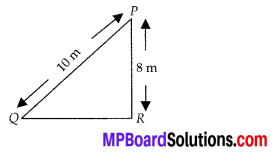⇒ PQ = 10 m, PR = 8 m
Now, in the right ∆PQR, PQ2 = PR2 + QR2
⇒ 102 = 82 + QR2
[using Pythagoras theorem]
⇒ QR2 = 102 – 82 = (10 + 8)(10 – 8)
= 18 × 2 = 36
QR = $$\sqrt{36}$$ = 6m
Thus, the distance of the foot of the ladder from the base to the wall is 6 m.

Question 10.
A guy wire attached to a vertical pole of height 18 m is 24 m long and has a stake attached to the other end. How far from the base of the pole should the stake be driven so that the wire will be taut?
Solution:
Let AB is the wire and BC is the vertical pole. The point A is the stake.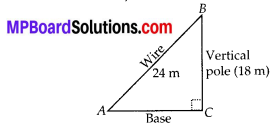Now, in the right AABC, using Pythagoras Theorem, we have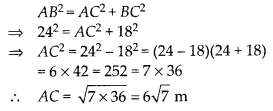Thus, the stake is required to be taken at $$6 \sqrt{7}$$m from the base of the pole to make the wire taut.Question 11.
An aeroplane leaves an airport and flies due north at a speed of 1000 km per hour. At the same time, another aeroplane leaves the same airport and flies due west at a speed of 1200 km per hour. How far apart will be the two planes after $$1 \frac{1}{2}$$ hours?
Solution:
Let the point A represent the airport. Plane-I fly towards North,
∴ Distance of the plane-I from the airport after $$1 \frac{1}{2}$$ hours = speed × time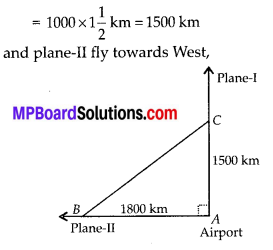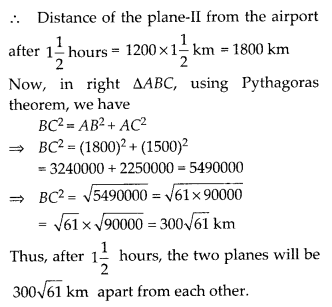Question 12.
Two poles of heights 6 m and 11 m stand on a plane ground. If the distance between the feet of the poles is 12 m, find the distance between their tops?
Solution:
Let the two poles AB and CD are such that the distance between their feet AC = 12m.
∵ Height of pole-1, AB = 11 m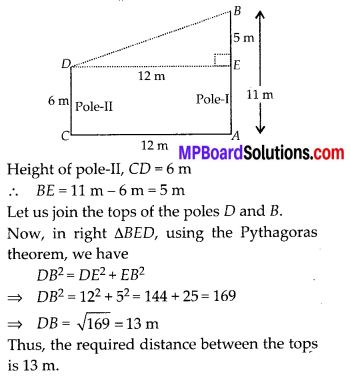Question 13.
D and E are points on the sides CA and CB respectively of a triangle ABC right angled at C. Prove that AE2 + BD2 = AB2 + DE2.
Solution:
We have a right ∆ABC such that ∠C = 90°.
Also, D and E are points on CA and CB respectively.
rain We have a right ∆ABC such that ∠C = 90°.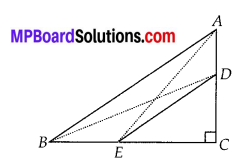Let us join AE and BD.
In right ∆ACB, using Pythagoras theorem
AB2 = AC2 + BC2 …… (1)
In right ∆DCE, using Pythagoras theorem,
DE2 = CD2 + CE2 …… (2)
Adding (1) and (2), we get
AB2 + DE2 = [AC2 + BC2] + [CD2 + CE2]
= AC2 + BC2 + CD2 + CE2 = [AC2 + CE2] + [BC2 + CD2] …. (3)
In right ∆ACE,
AC2 + CE2 = AE2 …… (4)
In right ∆BCD,
BC2 + CD2 = BD2 …….. (5)
From (3), (4) and (5), we have AB2 + DE2 = AE2 + BD2 or AE2 + BD2 = AB2 + DE2

Question 14.
The perpendicular from A on side BC of a ∆ABC intersects BC at D such that DB = 3CD (see figure). Prove that 2AB2 = 2AC2 + BC2.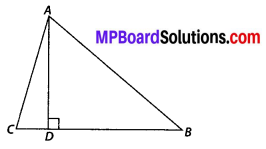Solution: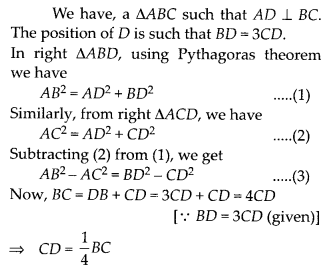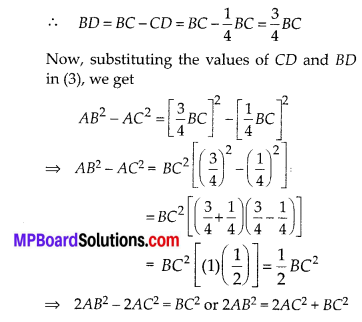Question 15.
In an equilateral triangle ABC, D is a point on side BC such that BD = $$\frac{1}{3}$$BC. Prove that 9AD2 = 7AB2.
Solution:
We have an equilateral ∆ABC; in which D is a point on BC such that BD = $$\frac{1}{3}$$ BC.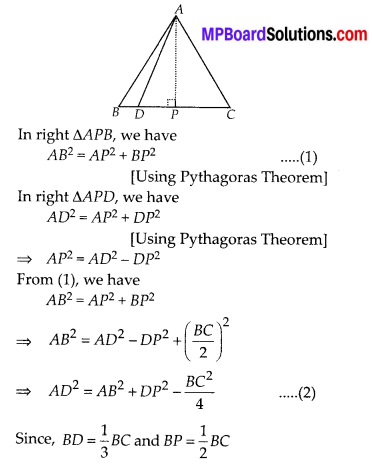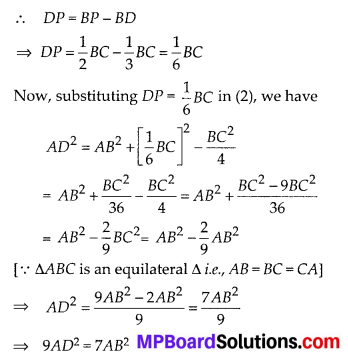Question 16.
In an equilateral triangle, prove that three times the square of one side is equal to four times the square of one of its altitudes.
Solution:
We have an equilateral ∆ABC, in which AD ⊥ BC.
Since, an altitude in an equilateral A, bisects the corresponding side.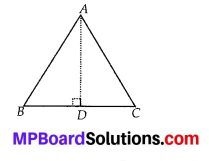∴ D is the mid-point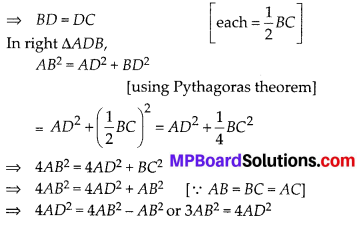Question 17.
Tick the correct answer and justify : In ∆ABC, AB = $$6 \sqrt{3}$$ cm, AC = 12 cm and BC = 6 cm. The angle B is
(A) 120°
(B) 60°
(C) 90°
(D) 45°
Solution:
(C): We have, AB = $$6 \sqrt{3}$$ cm, AC = 12 cm, and BC = 6 cm